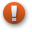# Gauge Theory of Economics

(diff) ← Older revision | Latest revision (diff) | Newer revision → (diff)This article is a stub. You can help us by editing this page and expanding it.
$$q = \frac{ {p_0}\cdot{q} }{ {p_0}\cdot{q_0} } q_0 + (q - \frac{ {p_0}\cdot{q} }{ {p_0}\cdot{q_0} } q_0)$$
Suppose that we live in a world where there are only 3 different types of items for sale: apples, berries and cherries (A, B and C respectively.) Say today we pick up our basket and go to the market. At the market, the price of each item is posted up as a number on the wall where we can see. So, we represent the prices by a $${1}\times{3}$$ row vector $$p$$. On the other hand, we buy different quantities of each item and so a $${3}\times{1}$$ column vector $$q$$ denotes the list of 3 quantities for items A, B and C.